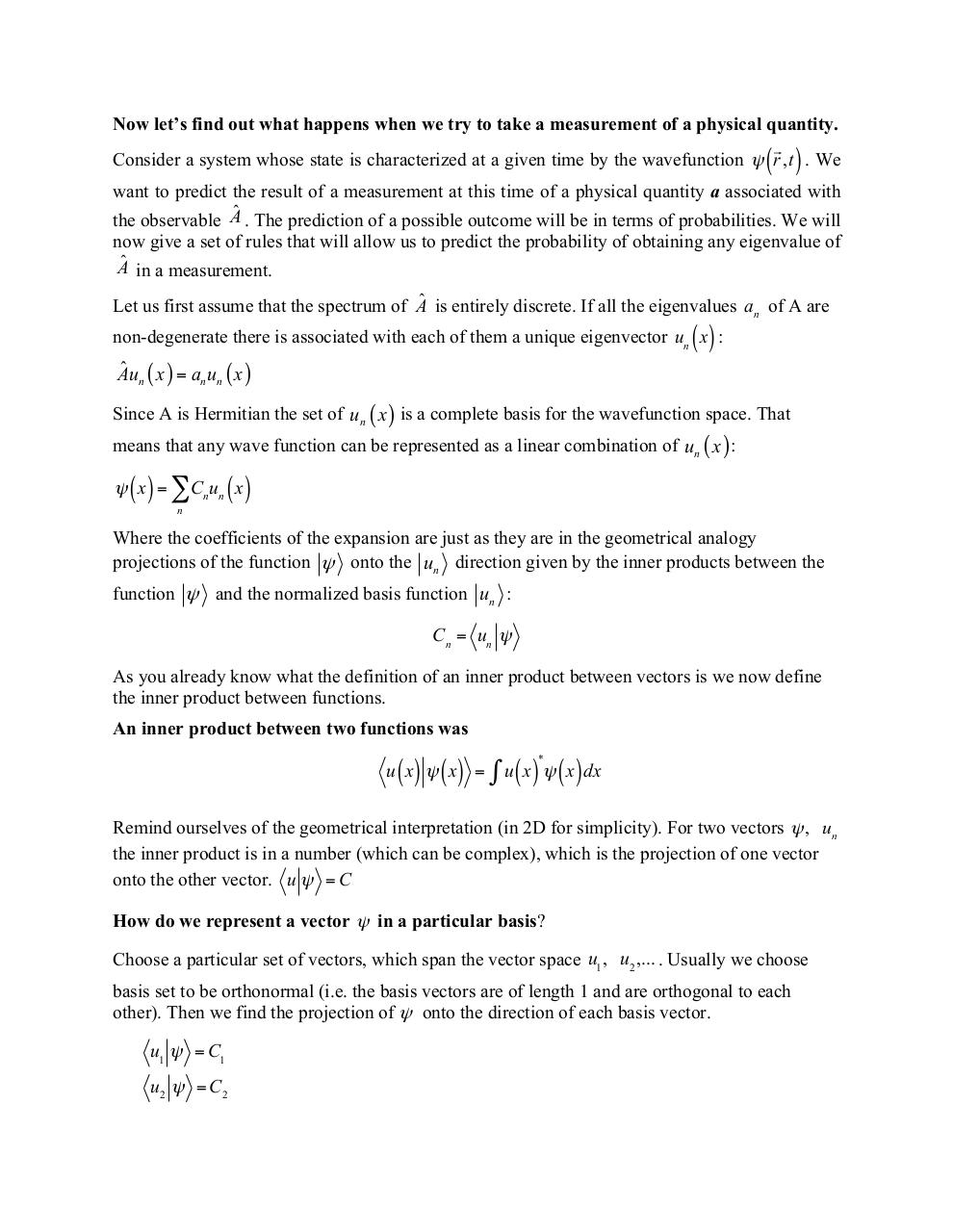# L7+QM+Measurements+and+Constants+of+Motion.pdfPage 1 2 3 4 5 6 7 8 9

#### Text preview

Now let’s find out what happens when we try to take a measurement of a physical quantity.

Consider a system whose state is characterized at a given time by the wavefunction ψ r ,t . We

( )

want to predict the result of a measurement at this time of a physical quantity a associated with
the observable Aˆ . The prediction of a possible outcome will be in terms of probabilities. We will
now give a set of rules that will allow us to predict the probability of obtaining any eigenvalue of
Aˆ in a measurement.
Let us first assume that the spectrum of Aˆ is entirely discrete. If all the eigenvalues an of A are

()

non-degenerate there is associated with each of them a unique eigenvector un x :

ˆ ( x) = a u ( x)
Au
n
n n
Since A is Hermitian the set of un ( x ) is a complete basis for the wavefunction space. That
means that any wave function can be represented as a linear combination of un ( x ) :

ψ ( x ) = ∑Cn un ( x )
n

Where the coefficients of the expansion are just as they are in the geometrical analogy
projections of the function ψ onto the un direction given by the inner products between the
function ψ and the normalized basis function un :

Cn = un ψ
As you already know what the definition of an inner product between vectors is we now define
the inner product between functions.
An inner product between two functions was
*

( ) ( ) ∫ u ( x) ψ ( x) dx

u x ψ x =

Remind ourselves of the geometrical interpretation (in 2D for simplicity). For two vectors ψ , un
the inner product is in a number (which can be complex), which is the projection of one vector
onto the other vector. u ψ = C
How do we represent a vector ψ in a particular basis?
Choose a particular set of vectors, which span the vector space u1 , u2 ,... . Usually we choose
basis set to be orthonormal (i.e. the basis vectors are of length 1 and are orthogonal to each
other). Then we find the projection of ψ onto the direction of each basis vector.

u1 ψ = C1
u2 ψ = C2